# Alignment Method for an Off-Axis Paraboloid

## 1. Geometry

The geometry of an off-axis section of a paraboloid is schematically illustrated in Figure 1a (below):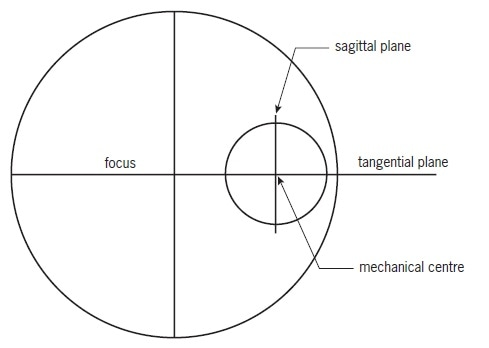Figure 1a. Definition of tangential and sagittal planes in relation to the geometry of an off-axis paraboloid.

The tangential plane can be seen passing through the mechanical center of the off-axis section and the optical axis. While perpendicular to the tangential plane, the sagittal plane also crosses through the mechanical center of the mirror as well as the focus of the parabola.

Figure 1b (below), shows a cross-section through the mirror in the tangential plane with all significant parameters displayed.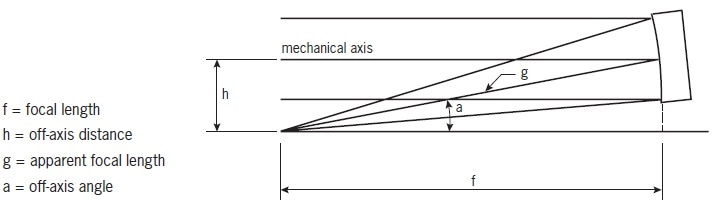Figure 1b. Important parameters that characterise an OAP.

The determining relationships between these parameters are: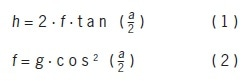Where:

f: is the true focal length (i.e. distance between vertex and focus)
g: is apparent focal length (i.e. distance between mechanical center and focus)
a: is the off-axis angle

Equation (1) illustrates the relationship between the off-axis distance, h, and the off-axis angle, a.

Equation (2) illustrates the relationship between the true focal length, f, and apparent focal length g, at a given off-axis angle.

## 2. Initial Alignment

Once the manufacturing process has been completed, the parameters of the off-axis parabolic mirror are determined, and a fiducial mark inscribed on the edge to denote the direction of the vertex (see Figure 2). Thus, the arrow points directly toward the optical axis.

Initial alignment is typically deployed using these measured parameters to set the mirror in approximate adjustment. Notably, this procedure is subject to the routine mechanical imprecisions of both setting and measurement.

Thus, the adjustment at this stage may not be adequate or precise enough to produce  an ideal performance in the mirror.

## 3. Types of Errors

The prime method to abolish the remaining alignment error is by use of an interferometer as demonstrated in Figure 2. This can be done using the Optical Surfaces Ltd. Scatterplate, or LUPI interferometers, or even a Fizeau type interferometer such as those made by Zygo, Wyko, Müller Wedel, etc. Using a plane mirror to reflect the collimated light from the parabola back to the common focus of the interferometer and parabola.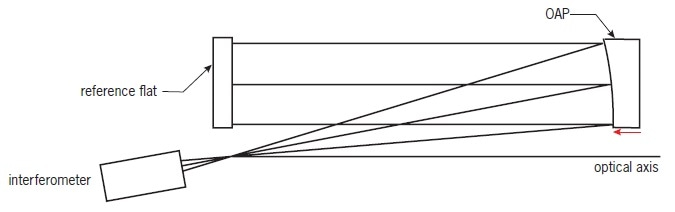Figure 2. Interferometric set-up.

During this type of testing, the mirror is used twice, or in a “double pass”. Hence, any errors witnessed are 2x the actual wavefront errors of the mirror under test, and 4x the surface errors.

The wavefront error calculated by the interferometer may be regarded as indicating the alignment errors. These errors are of three main types:

1. Focus and tilt
2. Incorrect setting of off-axis distance
3. Incorrect setting in tangential plane

### i). Focus and Tilt

These errors can be easily understood as indicated by the appearance of circular or elliptical fringes in the case of focus. Tilt errors are indicated by straight fringes or a shift of the center of the fringe pattern indicate.

As a guide to the sensitivity to a focal shift the equation below may be used.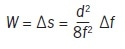Where:

f is the focal length
∆s is the resultant change of sagittal depth of the reference sphere
∆f is the focal shift
W is the wavefront error
d is the diameter of the mirror

### ii). Incorrectly Set Off-Axis Distance

In the event of setting the off-axis distance incorrectly (equivalent to an incorrect setting of the off-axis angle) then a comatic error can be observed. This error will appear as an astigmatism (at least for small misalignments), after all, we are contending with an off-axis section of an axially symmetric parabolic mirror

Light from the tangential and sagittal planes will thereby focus at varying points on the optical axis. Common interferograms are shown in figure 3. Figure 3a demonstrates an example at best focus. Figures 3b and 3c illustrate the effect of defocus. Note the characteristic elliptical fringes with the major/minor axis of the ellipse parallel to the tangential plane.

Again, as an indication of the magnitude of the wavefront error due to coma, the following equation may be used: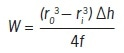Where:

r0 is the distance between the optical axis and the outside edge of the mirror (i.e. half the diameter of the parent parabola)
ri is the distance between the optical axis and the inside edge of the mirror.
f is the true focal length
∆h is the error of setting of the axial displacement
W is the wavefront error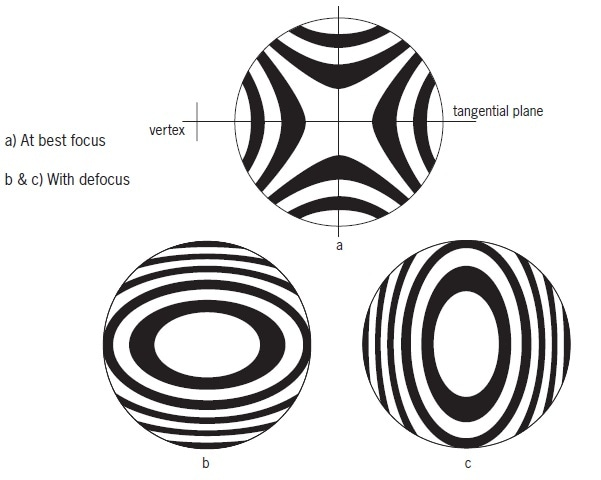Figure 3. Interferograms due to incorrect setting of off-axis distance.

### iii). Incorrect Alignment of the Mirror in Rotation

These kinds of errors occur due to the mirror’s rotational misalignment about its mechanical center. The result is the shifting of the mirror’s “true optical axis” out of the tangential plane as shown in Figure 4. As above, this error is comatic in nature. Yet, since this is an out of plane error, the coma is inclined at 45° to the tangential plane.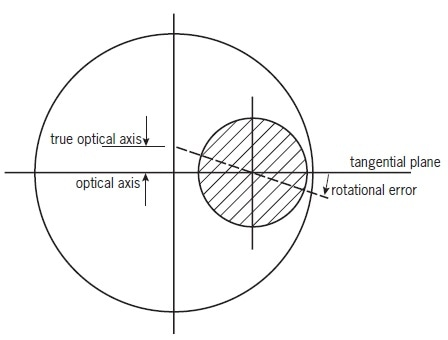Figure 4. Misalignment due to rotation of sector about its mechanical centre. The fiducial marks on the mirror correspond to the dashed line.

This kind of error is clearly illustrated in Figure 5. Additionally, the example shown in Figure 5a demonstrates best focus and the subsequent images (b, c, d, & e) display the effect of defocus and tilt. Once again, note the typical elliptical fringes when defocused – the major/minor axis is inclined at 45°.

To calculate the wavefront error due to this rotational misalignment the following equation may be used: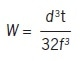Where:

f is the focal length
d is the diameter of the mirror
t is the distance between the “true” focus and the tangential planeFigure 5. Interferograms due to rotational error about the mechanical centre of the mirror.

### iv). Mixed Errors

Figure 6 is illustrative of the resultant interferograms when a system contains a mixture of all error types.

With defocus, the fringes still demonstrate an elliptical nature but now there is no specific direction in which the major/minor axis point – its direction depends on a certain mixture of the error types. Such interferograms are to be found in a mirror which has been aligned with little care or attention.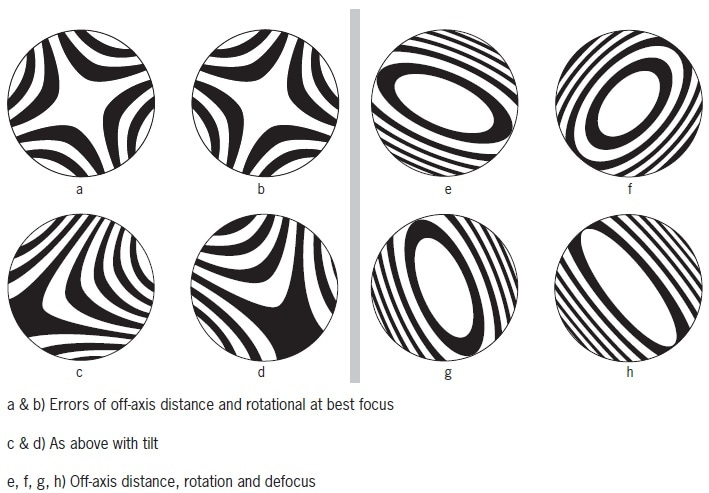Figure 6. Interferograms due to a combination of alignment errors.

## 4. Final Alignment – Step by Step Instructions

### Step 1:

In order to perfect the alignment for the desired performance it is best to eliminate each type of error in turn. Prior to the action, it is simple enough to deliberately defocus the system (if it is not so already). Then, it should be possible to attain the fringes of the type shown in Figures 7a, 8a (The direction of the major axis of the elliptical fringes is not important and may indeed be ≈ 90° to those shown – the direction is a function of the magnitude and sense of the errors).

### Step 2:

This should begin with rotational errors by rotating the mirror about its mechanical center. This will change the position angle of the axis of the elliptical fringes as well as the eccentricity of these fringes. The correct direction of rotation may easily be resolved by an inspection of the fringes, since the correct sense will minimize ellipse eccentricity. The effect can be seen in Figures 7a-d, and 8a-d. Note, the major axis of the fringes may rotate clockwise or anticlockwise depending on the nature of the combination of error types.

### Step 3:

This rotation should be continued until the major axis of the fringes is either perpendicular or parallel to the tangential plane (Figures 7d, 8d). If rotating the mirror is a task too complex to undertake, the same effect may be achieved in the following way – change the “height” of the interferometer (movement in the sagittal plane) and resolve the image to the interferometer by tilting the mirror or reference flat appropriately. Thus, no errors of mirror rotation are apparent within the system.

### Step 4:

The next phase calls for the elimination of errors due to off-axis setting. By either rotating the mirror about its vertical axis through the mechanical center of the mirror and perpendicular to the tangential plane or laterally displacing the mirror. Then, the correct direction can be easily determined by an inspection of the fringes – the correct direction will reduce the eccentricity. When the fringes are circular (Figures 7e and 8e) the system is fully aligned, and it is now only necessary to correctly focus the system.

### Step 5:

If the initial alignment results in a mirror with a significant amount of misalignment the above procedure may need to be carried out more than once – thereby, reducing the errors on each successive iteration.

Some errors may remain after optimizing the mirror alignment. These will most-likely relate to residual errors in the mirror surface and should be comparable to those in the interferogram supplied with the mirror.

As previously noted, the perceived fringes are four times as sensitive as the surface error measurement by which the mirror was identified. If errors that cannot be accounted for are present, this may be due to the test flat, distortion of either mirror caused by the mount or errors in the interferometer.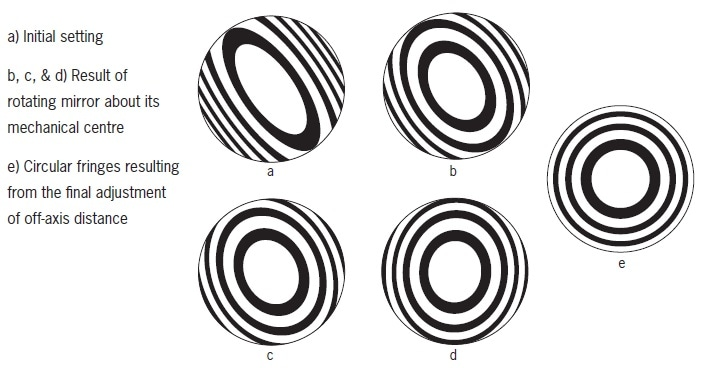Figure 7. Typical interferograms seen during alignment.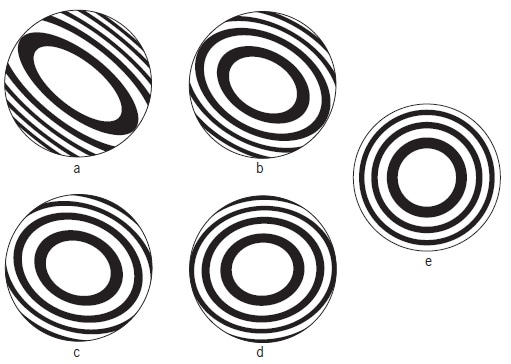Figure 8. As Figure 7 but with a different combination of error types. Compare the direction of rotation of the major axis of the elliptical fringes.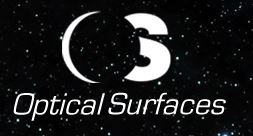This information has been sourced, reviewed and adapted from materials provided by Optical Surfaces Ltd.

## Citations

• APA

Optical Surfaces Ltd.. (2020, March 11). Alignment Method for an Off-Axis Paraboloid. AZoOptics. Retrieved on September 26, 2020 from https://www.azooptics.com/Article.aspx?ArticleID=1675.

• MLA

Optical Surfaces Ltd.. "Alignment Method for an Off-Axis Paraboloid". AZoOptics. 26 September 2020. <https://www.azooptics.com/Article.aspx?ArticleID=1675>.

• Chicago

Optical Surfaces Ltd.. "Alignment Method for an Off-Axis Paraboloid". AZoOptics. https://www.azooptics.com/Article.aspx?ArticleID=1675. (accessed September 26, 2020).

• Harvard

Optical Surfaces Ltd.. 2020. Alignment Method for an Off-Axis Paraboloid. AZoOptics, viewed 26 September 2020, https://www.azooptics.com/Article.aspx?ArticleID=1675.